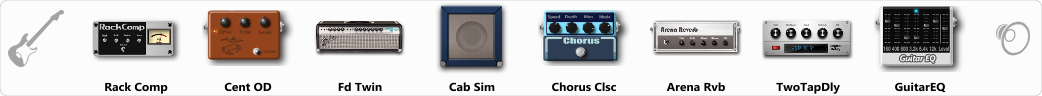# worship drive

Discussion in 'ToneLib-GFX presets' started by blayde55, Jun 26, 2019.

1. worship drive

Preset name: worship drive

drive signal chain for praise and worship

Effects chain:Effect: "Rack Comp" (Dynamics / Filter), active - "yes"
"Threshold (dB)" = -19
"Ratio" = 5
"Attack" = Fast
"Release (ms)" = 250
"Knee" = 25
"Level (dB)" = 7

Effect: "Cent OD" (Overdrive / Distortion), active - "yes"
"Drive" = 42
"Tone" = 82
"Level" = 43

Effect: "Fd Twin" (Amp simulators), active - "yes"
"Gain" = 50
"Bass" = 50
"Middle" = 50
"Treble" = 50
"Presence" = 50
"Master" = 50
"Output" = 50
"Level (dB)" = 0

Effect: "Cab Sim" (Cabinets), active - "yes"
"Model" = 4x12" 1960 T75
"Level (dB)" = 0

Effect: "Chorus Clsc" (Modulation / Sfx), active - "yes"
"Speed" = 5.2
"Depth" = 58
"Center" = 3.3
"Mode" = Mono

Effect: "Arena Rvb" (Reverb), active - "yes"
"Time" = 4.6
"PreDelay" = 23
"LoDamp" = 18
"HiDamp" = 21
"Mix" = 24

Effect: "TwoTapDly" (Delay), active - "yes"
"Time" = 756
"Feedback" = 44
"Tone" = 76
"Sens" = 0
"TapTime" = 5.0
"Mix" = 49

Effect: "GuitarEQ" (Dynamics / Filter), active - "yes"
"160 Hz" = 0
"400 Hz" = -5
"800 Hz" = 0
"1.6 kHz" = 0
"3.2 kHz" = 0
"6.4 kHz" = 4
"12 kHz" = 5
"Level (dB)" = 0

Note: You will need to download and install the ToneLib-GFX software to use the preset.

File size:
684 bytes
Views:
5,782